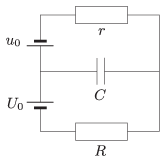Mathematical and Physical Journal
for High Schools
Issued by the MATFUND Foundation
 Already signed up? New to KöMaL?

#Problem P. 4730. (April 2015)

P. 4730. The terminal voltages across the voltage supplies connected as shown in the figure are constant. The voltage across the capacitor $\displaystyle C$ is $\displaystyle 1.8\,U_0$. If the terminals of the voltage supply $\displaystyle U_0$ is swapped over then the voltage across the capacitor decreases to $\displaystyle 1.4\,U_0$. Determine the ratios of $\displaystyle r/R$ and of $\displaystyle u_0/U_0$.(4 pont)

Deadline expired on May 11, 2015.

### Statistics:

 30 students sent a solution. 4 points: Balogh Menyhért, Bekes Nándor, Berta Dénes, Blum Balázs, Bugár 123 Dávid, Csorba Benjámin, Fehér Balázs, Fekete Balázs Attila, Fekete Panna, Fülöp Erik, Iván Balázs, Kasza Bence, Marosvári Kristóf, Molnár 128 Szilárd, Molnár Elizabet, Nagy 555 Botond, Németh Flóra Boróka, Olosz Balázs, Sal Kristóf, Szántó Benedek, Szentivánszki Soma , Tóth Bence Tamás, Varga-Umbrich Eszter, Wiandt Péter, Zöllner András. 2 points: 2 students. 1 point: 1 student. 0 point: 2 students.

Problems in Physics of KöMaL, April 2015